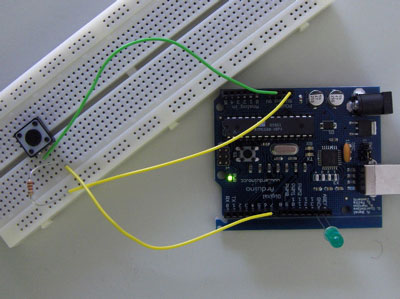Examples > Digital I/O

## Switch

This example demonstrates the use of a pushbutton as a switch: each time you press the button, the LED (or whatever) is turned on (if it's off) or off (if on). It also debounces the input, without which pressing the button once would appear to the code as multiple presses.

### Circuit

A push-button on pin 2 and an LED on pin 13.### Code

```/* switch
*
* Each time the input pin goes from LOW to HIGH (e.g. because of a push-button
* press), the output pin is toggled from LOW to HIGH or HIGH to LOW.  There's
* a minimum delay between toggles to debounce the circuit (i.e. to ignore
* noise).
*
* David A. Mellis
* 21 November 2006
*/

int inPin = 2;         // the number of the input pin
int outPin = 13;       // the number of the output pin

int state = HIGH;      // the current state of the output pin
int previous = LOW;    // the previous reading from the input pin

// the follow variables are long's because the time, measured in miliseconds,
// will quickly become a bigger number than can be stored in an int.
long time = 0;         // the last time the output pin was toggled
long debounce = 200;   // the debounce time, increase if the output flickers

void setup()
{
pinMode(inPin, INPUT);
pinMode(outPin, OUTPUT);
}

void loop()
{

// if the input just went from LOW and HIGH and we've waited long enough
// to ignore any noise on the circuit, toggle the output pin and remember
// the time
if (reading == HIGH && previous == LOW && millis() - time > debounce) {
if (state == HIGH)
state = LOW;
else
state = HIGH;

time = millis();
}

digitalWrite(outPin, state);